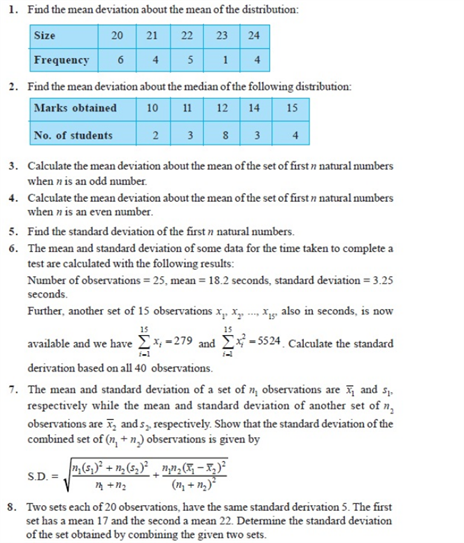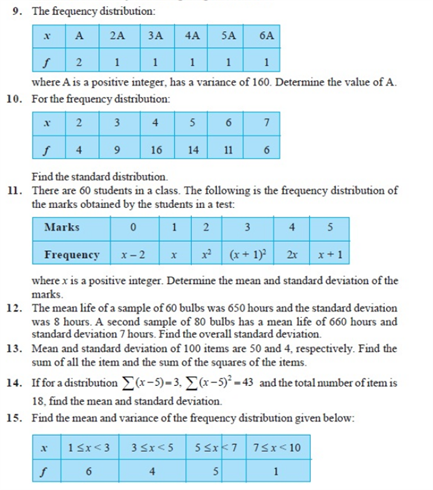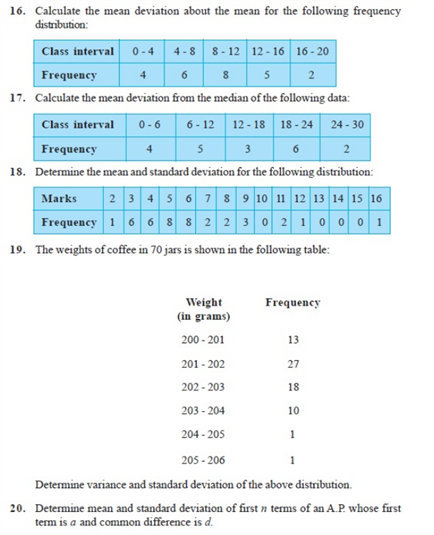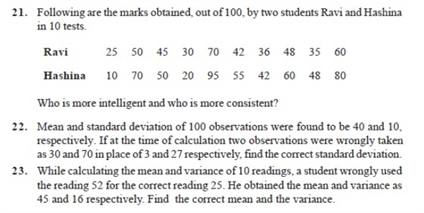# Chapter 15 - Statistics#### Practise This Question

The mean of a set of  numbers is ¯¯¯x If each number is multiplied by λ , then the mean of new set is